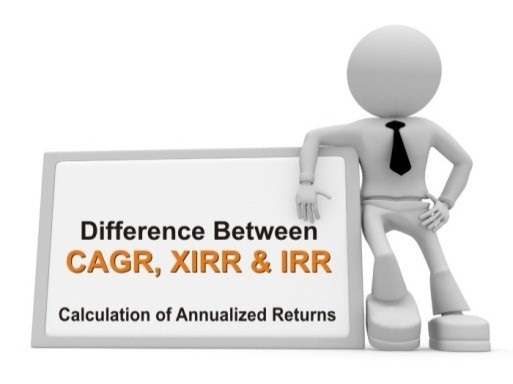Blog DetailAMFI-Registered Mutual Fund Distributor# Page Title### Understanding Return

December 5,2018

Understanding Return

Calculating return would have been easier, if we had been investing exactly for one year. But that doesn’t happen in practical world. Investment is normally done in staggered manner and each investment is not kept for same period of time. Withdrawal also might happen over a period of time.

To compare the return from various investment plans, it is necessary to have a common parameter which can be used for all types of investments with different investment amounts and different holding period. That common parameter is to assume that all investment returns get compounded annually.

If investment is held for lesser  than one year, then we need to calculate the return in percentage terms by assuming that the investment is held for one year.

CAGR – Compounded Annual Growth Rate

If you want to calculate the return for one time investment then CAGR (Compounded Annual Growth Rate) is used. But when the investment is done  periodically or staggered over a period of time, CAGR is not useful to calculate the return.

In case of staggered investment, either IRR or XIRR can be used.

IRR – Internal Rate of Return

If the investment is done in strict periodic manner, you may use IRR to find out the rate of return. For example if investment is done at fix interval (Monthly/quarterly/yearly) and withdrawal  only at the end of the entire tenure, IRR can be used to find out the return.

XIRR

If cashflow includes frequent inflow as well as outflow over a period of time, we need to use XIRR for calculating the rate of return. XIRR gives you the flexibility to assign specific dates for each  cash flow, making it a much more accurate calculation.

Though Return is one of the most important criteria but we should also look at other parameters like consistency, portfolio quality, risk, risk adjusted returns etc.

Tags :

All Blog